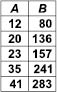# User ForumSubject :IMO    Class : Class 5

Which of the following rules could be used to describe the relationship between A and B ?(a) Subtract 7 from the value of A and then multiply by 4.
(b) Subtract 4 from the value of A and then multiply by 7.
(c) Multiply the value of A by 4 and then subtract 7.
(d) Multiply the value of A by 7 and then subtract 4.

D

Class : Class 6
D)